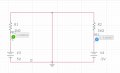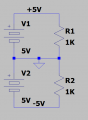# Negative voltage without affecting a microcontroller

Joined Mar 4, 2020
41
Hi, I'm sorry for the basic question but I'm trying to wrap my head around negative voltages.
I'm planning on creating a low pass filter on a signal that oscillates between +5 and -5V.
From the same +5 supply, I will have a microcontroller attached. I am trying to understand if the -5V circuit will cause problems for the microcontroller since at some point, the circuit between -5 and ground will be closed. I've tried to simplify the circuit but I'm not sure if it is really equivalent to what I wanna do.So basically, R1 would be my microcontroller. and R2 would be kinda like the load attached to my oscillating signal. Does that make sense?
The simulation shows that everything is alright. But I don't understand why current would flow from +5 to ground instead of +5 to -5. If that was the case, then that would probably break the microcontroller (or in this diagram, it would submit R1 to 10v). But this is not the case.
Can someone explain why that is?
And could you confirm that it is perfectly safe to have a uC in a circuit like that where a +/-5V signal would share the same ground?

Thank you.

#### ronsimpson

Joined Oct 7, 2019
2,095
Does it help do draw the schematic like this?
Battery 1 and R1 form a loop with current.
Battery 2 and R2 from a loop with current.
In a bigger picture Batteries 1 & 2 and R1,2 makes a big loop with no current in the ground area. In the center of the picture, in "ground", 5mA of current flows to the right and a different 5mA flows to the left. +5mA plus -5mA = 0mA.Joined Mar 4, 2020
41
Yes, that does help @ronsimpson . But in that case it's because both resistors are the same. So if I change R2 to 100ohm, it would yield a total of -45mA. But I guess that this current never flows through the top part of the circuit

#### ronsimpson

Joined Oct 7, 2019
2,095
If R2=100 then that current flows in R2 and R1=1k=5mA in the top half. You are right that the middle sees 45mA.

In some countries, the power company sends 110V, ground (neutral), and 110V. Much like your circuit. But there are long wires from the power source to the load. At my house, I have 110v or 220V much like you have options of 5V or 10V. A light bulb does not care if it is on +5V or -5V. In my house half of the power is connected to V1 and half to V2. (line1 and line 2) There is little current in the center wire because the loads are almost the same. The big loads are at 220 which cause no current in the center.

I hope this did not make more confusion.
ron simpson

#### BobTPH

Joined Jun 5, 2013
4,950
The top part of the circuit is connected to the bottom half in only 1 place, so they cannot affect each other.

Bob